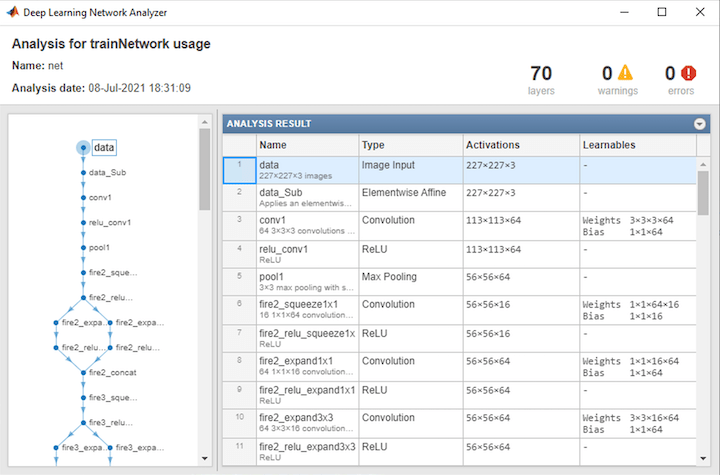# importONNXNetwork

Import pretrained ONNX network

## Syntax

``net = importONNXNetwork(modelfile,'OutputLayerType',outputtype)``
``net = importONNXNetwork(modelfile,'OutputLayerType',outputtype,'Classes',classes)``

## Description

````net = importONNXNetwork(modelfile,'OutputLayerType',outputtype)` imports a pretrained network from the ONNX™ (Open Neural Network Exchange) file `modelfile` and specifies the output layer type of the imported network.This function requires the Deep Learning Toolbox™ Converter for ONNX Model Format support package. If this support package is not installed, then the function provides a download link.```

example

````net = importONNXNetwork(modelfile,'OutputLayerType',outputtype,'Classes',classes)` additionally specifies the classes for a classification network.```

## Examples

collapse all

Download and install the Deep Learning Toolbox Converter for ONNX Model Format support package.

Type `importONNXNetwork` at the command line.

`importONNXNetwork`

If Deep Learning Toolbox Converter for ONNX Model Format is not installed, then the function provides a link to the required support package in the Add-On Explorer. To install the support package, click the link, and then click . Check that the installation is successful by importing the network from the model file `'cifarResNet.onnx'` at the command line. If the support package is installed, then the function returns a `DAGNetwork` object.

```modelfile = 'cifarResNet.onnx'; classes = ["airplane" "automobile" "bird" "cat" "dee" "dog" "frog" "horse" "ship" "truck"]; net = importONNXNetwork(modelfile,'OutputLayerType','classification','Classes',classes)```
```net = DAGNetwork with properties: Layers: [77×1 nnet.cnn.layer.Layer] Connections: [85×2 table]```

Import a residual neural network trained on the CIFAR-10 data set. Specify the file containing the ONNX network, its output type, and its output classes.

```modelfile = 'cifarResNet.onnx'; classes = ["airplane" "automobile" "bird" "cat" "deer" "dog" "frog" "horse" "ship" "truck"]; net = importONNXNetwork(modelfile, ... 'OutputLayerType','classification', ... 'Classes',classes)```
```net = DAGNetwork with properties: Layers: [77×1 nnet.cnn.layer.Layer] Connections: [85×2 table] ```

Analyze the imported network.

`analyzeNetwork(net)`## Input Arguments

collapse all

Name of ONNX model file containing the network, specified as a character vector or a string scalar. The file must be in the current folder, in a folder on the MATLAB® path, or you must include a full or relative path to the file.

Example: `'cifarResNet.onnx'`

Type of the output layer that the function appends to the end of the imported network, specified as `'classification'`, `'regression'`, or `'pixelclassification'`. Using `'pixelclassification'` appends a `pixelClassificationLayer` object (requires Computer Vision Toolbox™).

If a network in `modelfile` has multiple outputs, then you cannot specify the output layer types using this argument. Use `importONNXLayers` instead. `importONNXLayers` inserts placeholder layers for the outputs. After importing, you can find and replace the placeholder layers by using `findPlaceholderLayers` and `replaceLayer`, respectively.

Example: `'regression'`

Classes of the output layer, specified as a categorical vector, string array, cell array of character vectors, or `'auto'`. If `Classes` is `'auto'`, then the software sets the classes to `categorical(1:N)`, where `N` is the number of classes. If you specify a string array or cell array of character vectors `str`, then the software sets the classes of the output layer to `categorical(str,str)`.

Data Types: `char` | `categorical` | `string` | `cell`

## Output Arguments

collapse all

Pretrained network, returned as `DAGNetwork` object.

## Compatibility Considerations

expand all

Not recommended starting in R2018b

 Open Neural Network Exchange. https://github.com/onnx/.

 ONNX. https://onnx.ai/.